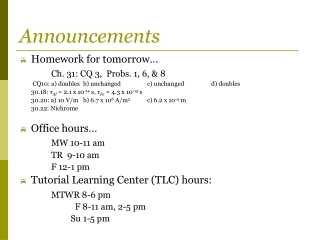Download PresentationAnnouncements

Announcements

Télécharger la présentationAnnouncements

- - - - - - - - - - - - - - - - - - - - - - - - - - - E N D - - - - - - - - - - - - - - - - - - - - - - - - - - -
Presentation Transcript

1. Announcements • Homework for tomorrow… Ch. 31: CQ 3, Probs. 1, 6, & 8 CQ10: a) doubles b) unchanged c) unchanged d) doubles 30.18:τAl = 2.1 x 10-14 s, τFe = 4.3 x 10-15 s 30.20: a) 10 V/m b) 6.7 x 106 A/m2 c) 6.2 x 10-4 m 30.22: Nichrome • Office hours… MW 10-11am TR 9-10 am F 12-1 pm • Tutorial Learning Center (TLC) hours: MTWR 8-6 pm F 8-11 am, 2-5 pm Su 1-5 pm

2. Chapter 31 Fundamentals of Circuits (Kirchoff’s Laws and the Basic Circuit & Energy and Power)

3. Review… • Kirchoff’s junction rule.. • Kirchoff’s loop rule…

4. i.e. 31.1:Two resistors and two batteries Analyze the circuit shown in the figure. • Find the current in and the potential difference across each resistor. • Draw a graph showing how the potential changes around the circuit, starting from V = 0V at the negative terminal of the 6 V battery. 4 Ω 6 V 9 V 2Ω

5. The Basic Circuit Consider the basic circuit shown...

6. The Basic Circuit Consider the basic circuit shown... Notice: • NO junctions, SAME I everywhere. • Assuming ideal wires (Rwire<<R).

7. Quiz Question 1 The potential difference across the 10 Ωresistor is… • 30 V. • 20 V. • 15 V. • 10 V. • 5 V.

8. Quiz Question 2 What things about the resistors in this circuit are the same for all three? • Current, I. • Potential difference, V. • Resistance, R. • 1. and 2. • 2. and 3.

9. Quiz Question 3 Which light bulb is brighter? • Light bulb A. • Light bulb B. • Both are the same brightness.

10. 31.3:Energy and Power Current is NOT used up by the light bulb, ENERGY is!

11. 31.3:Energy and Power Current is NOT used up by the light bulb, ENERGY is! Q: What is the rate at which the battery supplies energy to the charges?

12. 31.3:Energy and Power Current is NOT used up by the light bulb, ENERGY is! Q: What is the rate at which the battery supplies energy to the charges? A: SI Units?

13. 31.3:Energy and Power Current is NOT used up by the light bulb, ENERGY is! Q: What is the rate at which the battery supplies energy to the charges? A: SI Units? [P] = J/s or W

14. i.e. 31.2:Delivering Power A 90 Ω load is connected to a 120V battery. How much power is delivered by the battery?

15. 31.3:Energy and Power Pbat is the energy transferred per second from the battery’s store of chemicals to the moving charges that make up the current.

16. 31.3:Energy and Power Pbat is the energy transferred per second from the battery’s store of chemicals to the moving charges that make up the current. Q: Where does that energy go?

17. 31.3:Energy and Power Pbat is the energy transferred per second from the battery’s store of chemicals to the moving charges that make up the current. Q: Where does that energy go? A: • The battery’s chemical energy is transferred to the thermal energy of the resistors. • The rate at which the battery supplies energy is exactly equal to the rate at which the resistor dissipates energy!

18. i.e. 31.3:The power of light How much current is “drawn” by a 100 W light bulb connected to a 120 V outlet? What’s the resistance of the light bulb?

19. 31.3:Energy and Power Power dissipated by a resistor..

20. 31.3:Energy and Power Power dissipated by a resistor.. Notice: For resistors in series, the resistor with the largest resistance dissipates the most power. For resistors in parallel, the resistor with the smallest resistance dissipates the most power.

21. Quiz Question 4 Which resistor dissipates more power? • The 9  resistor. • The 1  resistor. • They dissipate the same power.